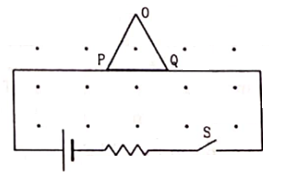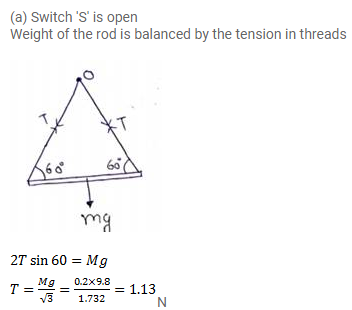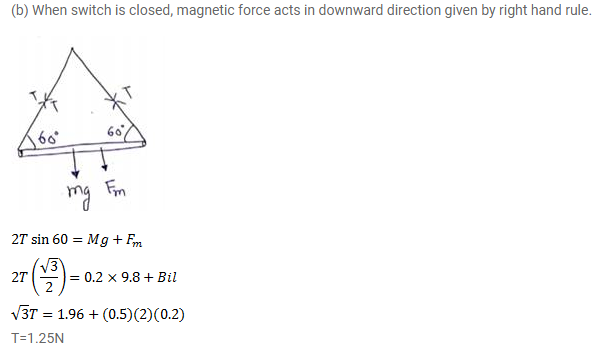# Figure shows a rod PQ of length 20.0cm and mass 200g

Question:

Figure shows a rod PQ of length $20.0 \mathrm{~cm}$ and mass $200 \mathrm{~g}$ suspended through a fixed point 0 by two threads of lengths $20.0 \mathrm{~cm}$ each. A magnetic field of strength $0.500 \mathrm{~T}$ exists in the vicinity of the wire PQ as shown in figure. The wires connecting $\mathrm{PQ}$ with the battery are loose and exert no force on $\mathrm{PQ}$.

(a) Find the tension in the threads when the switch $\mathrm{S}$ is open.

(b) A current of $2.0 \mathrm{~A}$ is established when the switch $\mathrm{S}$ is closed. Find the tension in the threads now.Solution: